+

# Elasticity

Author: Sophia Tutorial
##### Description:

Compare elasticity coefficients of different types of goods.

(more)### Developing Effective Teams

*No strings attached. This college course is 100% free and is worth 1 semester credit.

46 Sophia partners guarantee credit transfer.

299 Institutions have accepted or given pre-approval for credit transfer.

* The American Council on Education's College Credit Recommendation Service (ACE Credit®) has evaluated and recommended college credit for 33 of Sophia’s online courses. Many different colleges and universities consider ACE CREDIT recommendations in determining the applicability to their course and degree programs.

Tutorial
what's covered
This tutorial will cover the topic of elasticity, focusing on the three types of elasticity: own-price, cross-price, and income.

Our discussion breaks down as follows:

1. What Is Elasticity?
1. Perfectly Inelastic Demand
2. Inelastic Demand
3. Perfectly Elastic Demand
4. Elastic Demand
5. Unit Elastic Demand
2. Own-Price Elasticity
3. Cross-Price Elasticity
4. Income Elasticity
1. Normal Goods
2. Luxury Goods
3. Inferior Goods

## 1. What Is Elasticity?

Let's begin with a quick review of the laws of supply and demand:

As prices rise, consumers buy less and producers supply more.
As prices fall, consumers buy more and producers supply less.

In this tutorial, we will be discussing exactly how much more or less, and if it is the same for every good.

Elasticity is the measurement of the change in quantity demanded or supplied which indicates the sensitivity of one variable to characteristics of another variable or income.

In other words, how much do people respond--by buying or supplying more/less--when something else changes, like the price of the good itself, the price of a related good, or people's income?

The answer is that it really depends on the good or service:

• How necessary is it?
• Are there close substitutes?
• How expensive is it?
term to know
Elasticity
Measurement of change in quantity demanded (supplied) which indicates the sensitivity of one variable to characteristics of another variable or income

Let's explore some examples to illustrate the different measurements of elasticity.

1a. Perfectly Inelastic Demand
This is an extreme example of elasticity known as perfectly inelastic demand.

hint
Note, this example references demand because it is a bit easier to understand, but this could certainly apply to supply as well.

This reflects perfectly inelastic demand for a life-saving medicine. Notice that no matter what the price, this consumer is buying the exact same quantity--30 pills per month. Therefore, they are not responsive at all to a price change because a life-saving medicine is a necessity, and there are no substitutes.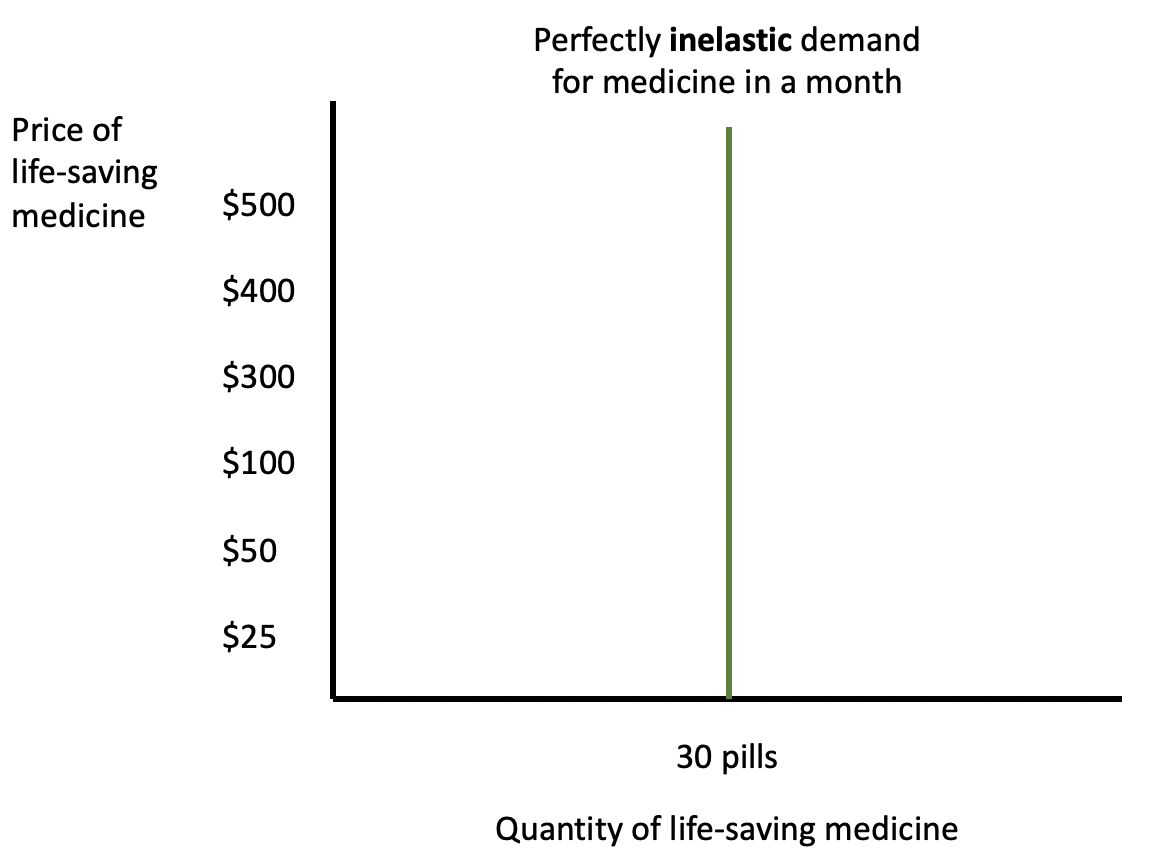hint
One way to remember the concept of inelasticity is by noting that the more straight up and down something is, it looks like the capital letter "I"--just like the graph above. Also, think about a product that is very inelastic, like insulin. Both words start with the same letters--"In"--which can also help you to remember the concept.

1b. Inelastic Demand
Now, most things in the world are not perfectly inelastic, but there are items like gasoline that are relatively inelastic.

This is not straight up and down, or completely vertical, because people do change their behavior somewhat as the price of gasoline goes up.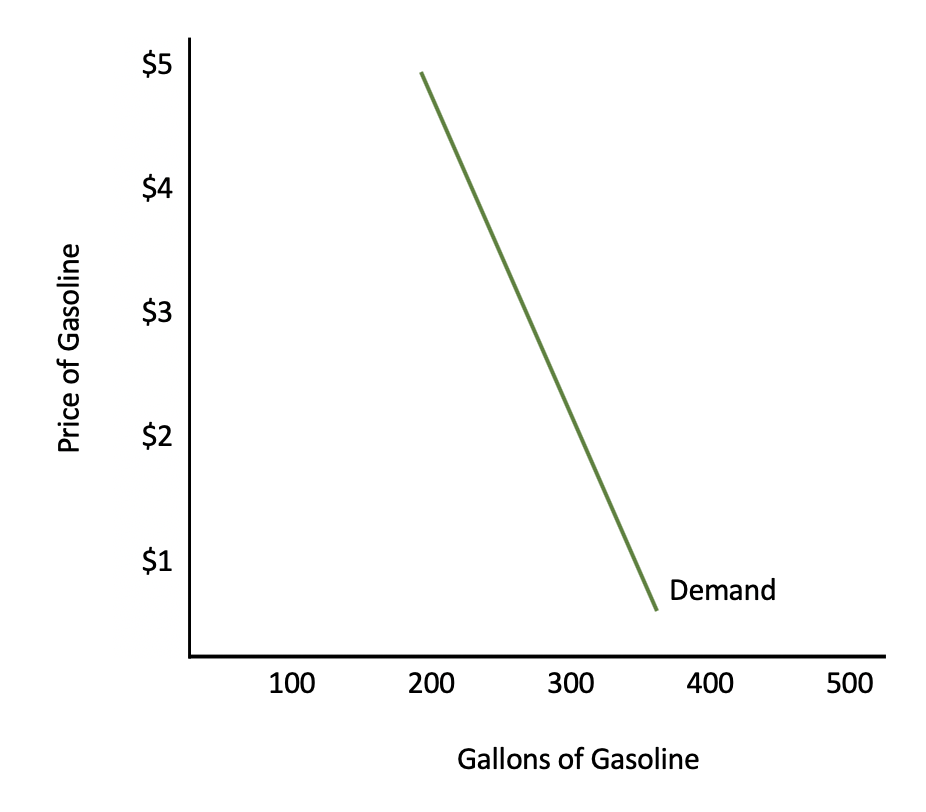However, notice how the price can change significantly, yet consumers do not change their purchasing habits very much. This is because most people view gasoline--at least in the short term--as a necessity.

big idea
The more vertical the curve, the more inelastic the demand or the supply for something is.

1c. Perfectly Elastic Demand
Now we shift to the other end of the spectrum, which is perfectly elastic demand.

We will use the example of a hot dog stand that is one of 200 stands in a baseball stadium. They are all selling an identical product and are all charging \$5 for that identical hot dog.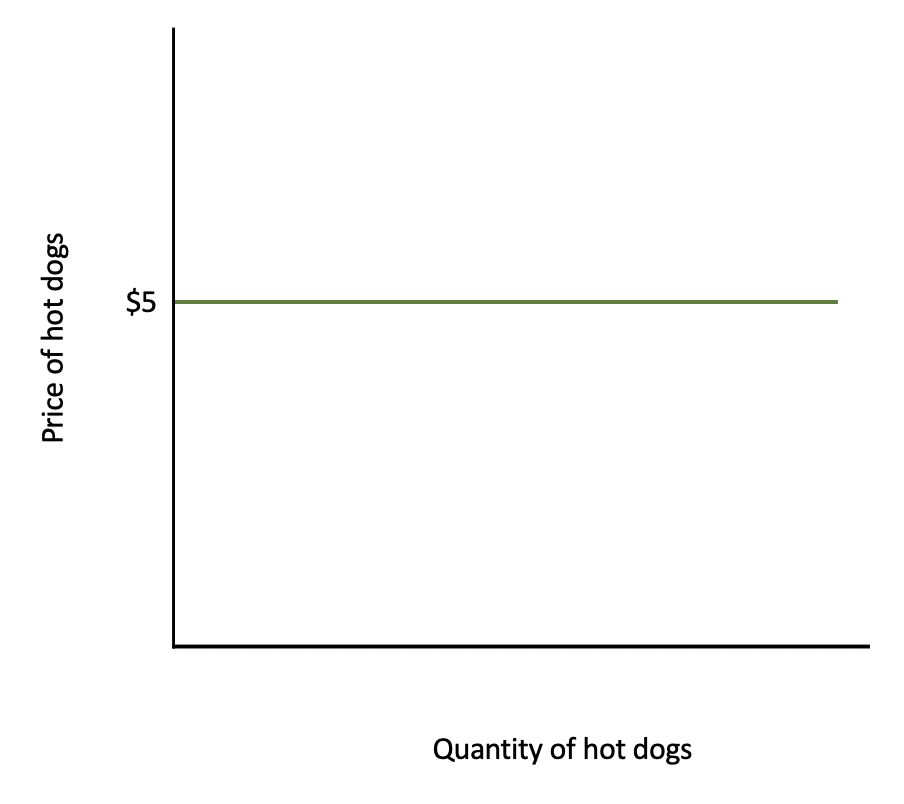If one hot dog stand out of 200 decided to raise price up to \$6, for instance, no one would purchase a hot dog there. The quantity demanded, in theory, according to this curve, would drop to zero, which is what a completely horizontal demand curve would imply--it is perfectly elastic.

hint
A visual way to remember the concept of perfectly elastic demand is if you place a line at the top of the graph, it looks like the letter "E," for elastic.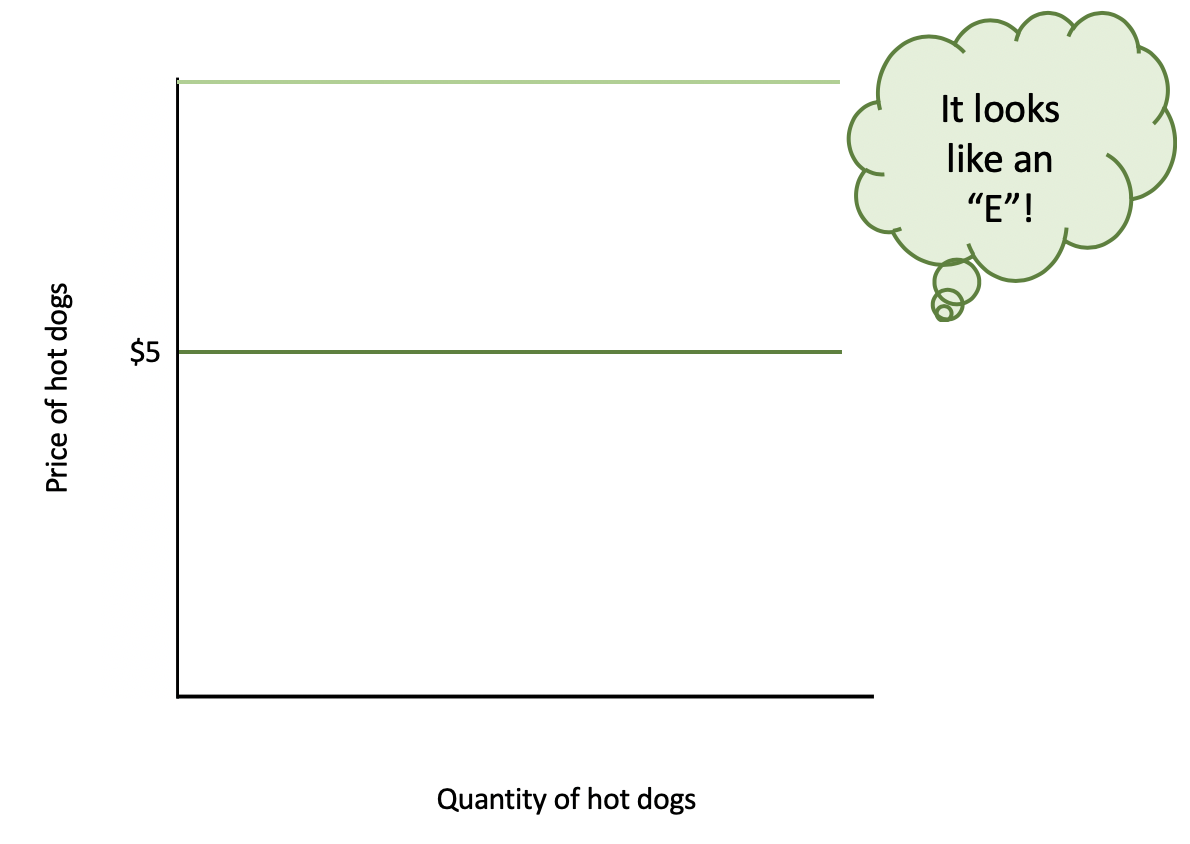1d. Elastic Demand
However, not many things are perfectly elastic in the world, so here is an example of something that is relatively elastic, which would be the demand for a specific brand of something.

Demand for a specific type of apples, like Gala apples, would be somewhat elastic.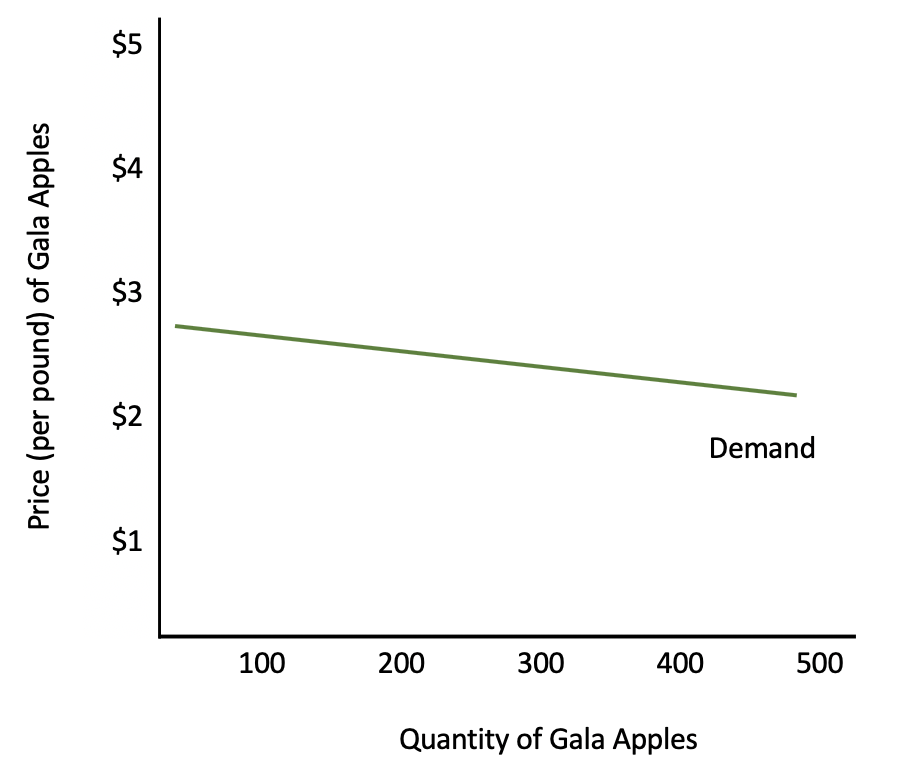It is not completely horizontal, because prices could increase a bit and you would still see purchases of Gala apples by people who simply prefer them.

However, quite a few people would change their minds. Notice how quantity is changing significantly compared to price because there are so many substitutes for Gala apples.

big idea
The more horizontal the curve, the more elastic it is going to be.

1e. Unit Elastic Demand
Finally, we have unit elastic demand, illustrated by this example using demand for chicken.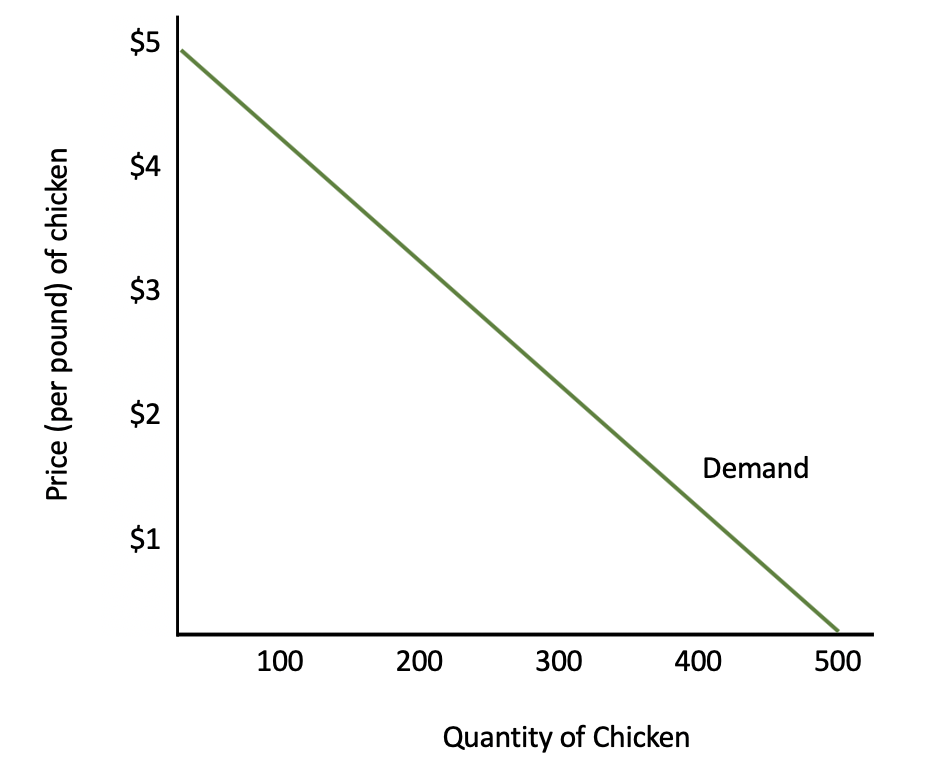Along this curve, let's assume that the price of chicken rises by 10%, and as a result, consumers buy exactly 10% less chicken. This means that the proportion of how much people respond is identical to the percentage change in price. In other words, people's response is proportional to the percentage change in price.

## 2. Own-Price Elasticity

All of these examples involve own-price elasticity, which is elasticity of either demand or supply when the price of a good changes, keeping all other variables and goods separate.

To fully understand the concept, let's walk through an example.

Is bottled water at the zoo elastic or inelastic in demand? Suppose the zoo was selling bottled water for \$2, and they were selling 500 bottles of water a day.

The zoo decides to raise prices to \$4 per bottle of water, and now they are selling 400 bottles of water a day.

The equation for elasticity is the percentage change in quantity-- how much people respond--divided by the percentage change in price.

formula
Elasticityhint
Keep in mind that for demand, elasticity will always be negative because the relationship between quantity and price is negative, as they move in opposite directions. For supply, it will always be positive because those two variables move in the same direction.

Elasticity Equation
For Demand For Supply
NEGATIVE, since Q & P move in opposite directions POSITIVE, since Q & P move in the same direction

For this example, we are only concerned about absolute value for own-price elasticity. We are simply looking at two points along the demand curve for water at the zoo to compare the change in quantity to the change in price (arc price elasticity).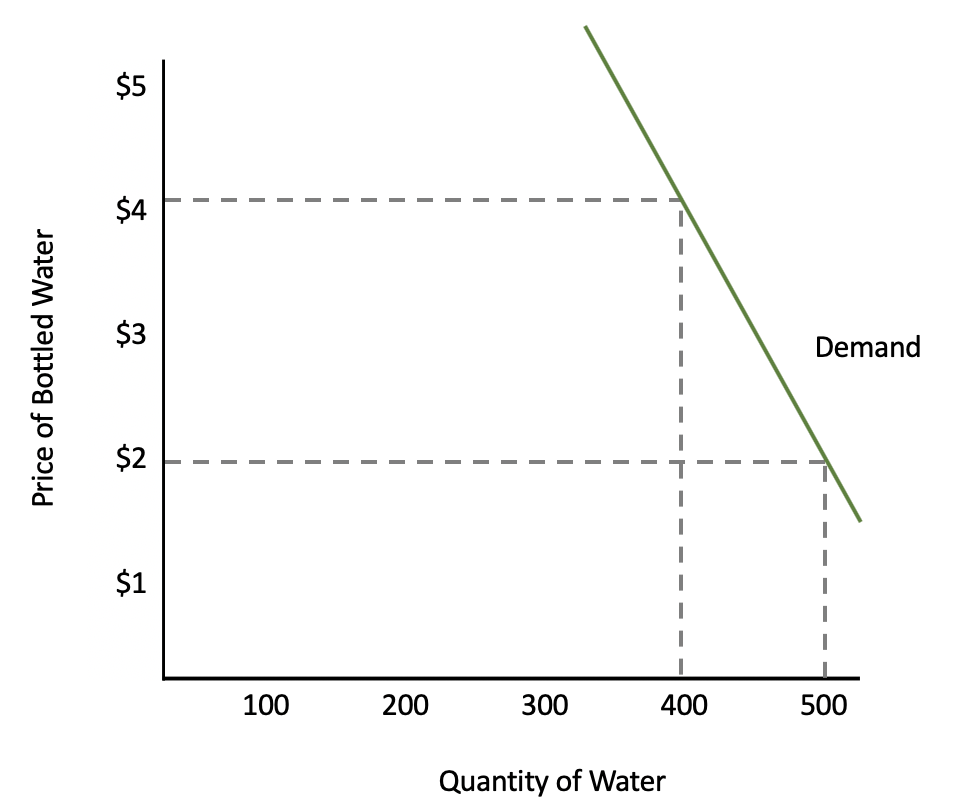Let's plug our numbers into the elasticity equation. Note that the absolute change from 500 bottles to 400 bottles is 100 bottles and the absolute change from \$2 to \$4 is \$2.Our elasticity coefficient is 0.20, but what does this number mean?

Well, when we look at the elasticity coefficient for own-price elasticity, a coefficient greater than 1 indicates that demand would be elastic because that would suggest that the numerator responds more than the denominator. So, people change their buying habits by more than the percentage change in price.

Inelastic demand results in a coefficient less than 1 because people are not as responsive to the change in price.

Unit elastic is when the two change proportionately, with an elasticity coefficient equal to 1.

term to know
Own-Price Elasticity
Elasticity of demand (supply) when the price of a good changes, keeping all other variables and goods separate

Price Elasticity Coefficients
E > 1 Elastic Demand/Supply
E < 1 Inelastic Demand/Supply
E = 1 Unit Elastic Demand/Supply

## 3. Cross-Price Elasticity

Now, cross-price elasticity is a change of demand or supply that occurs due to a change in the price of substitutes or complements.

To illustrate, let's look at an example using the brands Nike and Adidas. We are going to look at what will happen to the quantity of Nike, not when the price of Nike changes, but when the price of Adidas changes.

Quantity of Nike Shoes Price of Adidas Shoes
QA = 200 PA = \$110
QB = 240 PB = \$130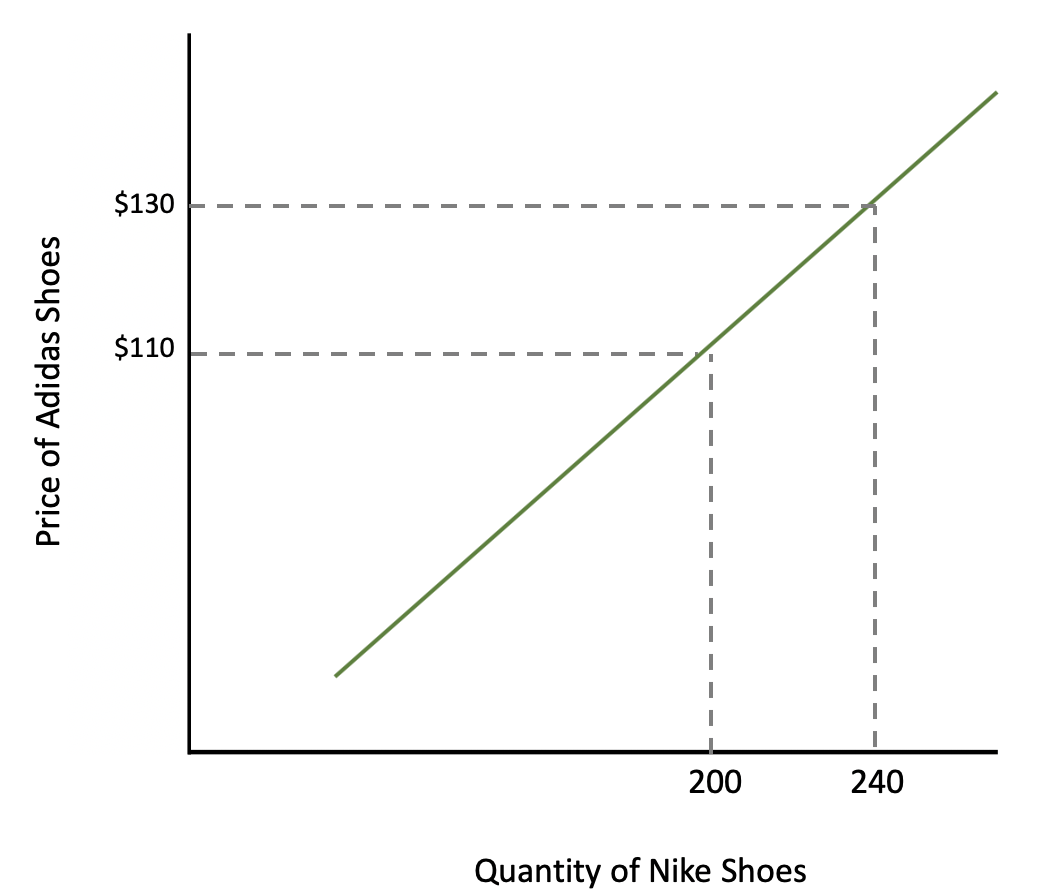Let's plug our numbers into the elasticity equation.If we perform the calculation, we get a cross-price elasticity of 1.1.

In this case, it does matter what direction price and quantity are moving. We are not just looking at the absolute value, because with cross-price elasticity:

• If the coefficient is positive--like in this example--then the two goods are substitutes.
• If the coefficient is negative, then the two goods are complements, meaning you would buy them together.

In this case, with a positive 1.11 coefficient, Nike and Adidas must be substitutes. When Adidas raises prices, people will buy more of a substitute, like Nike.

term to know
Cross-Price Elasticity
Change of demand (supply) that occurs due to change in price of substitutes or complements

Cross-Price Elasticity Coefficients
E < 0 Complements
E > 0 Substitutes

## 4. Income Elasticity

Finally, let's discuss our last type of elasticity, income elasticity. Income elasticity is an economic measure of change in relation to income and demand for normal, inferior, and luxury goods.

Continuing with Nike as an example, if our incomes rise, will we buy more or less Nike apparel?

For example, Tom's income increases from \$20,000 to \$40,000. He used to buy two pairs of Nike running shoes a year, but now he can afford to buy three pairs.

Quantity of Nike Shoes Income
QA = 2 Pairs IA = \$20,000
QB = 3 Pairs IB = \$40,000

Tom's demand curve is shown below. Notice, though, that instead of looking at what happens when the price changes, we are looking at what happens when someone's income changes.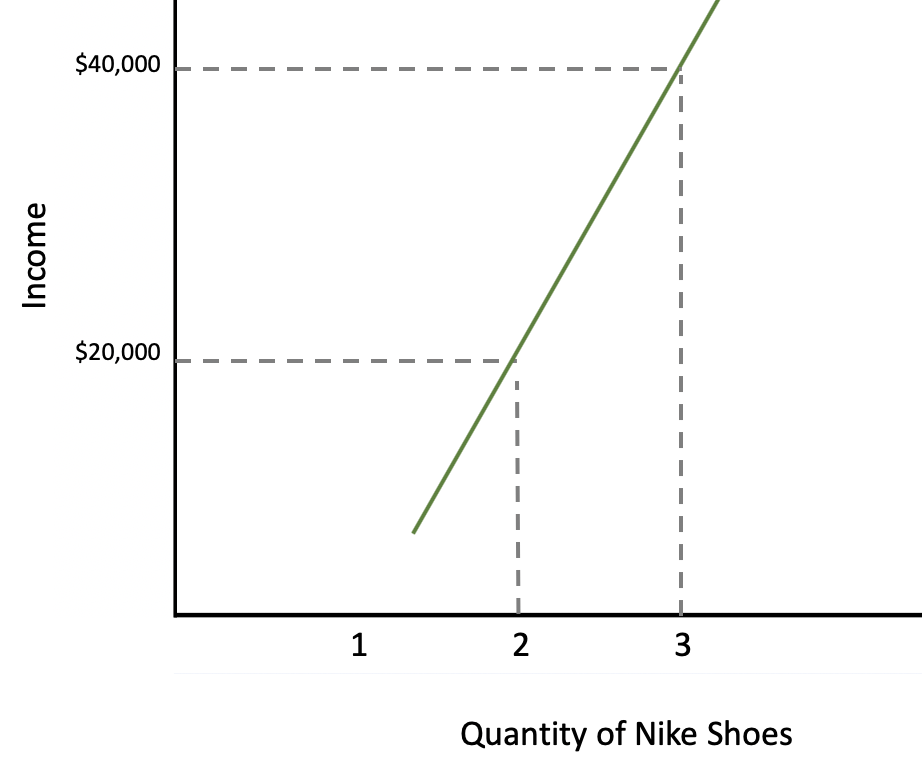As you can see, there is a positive relationship between income and Nike shoes purchased.

Let's plug our numbers into the elasticity equation.Performing the calculations, we see that we get an elasticity coefficient of a positive 0.50.

Again, the coefficient does matter in terms of the sign; it is not just absolute value. Therefore, that positive 0.50 tells us that the good is a normal good. If the coefficient were negative, the good would be an inferior good.

term to know
Income Elasticity
An economic measure of change in relation to income and demand for normal, inferior, and luxury goods

4a. Normal Goods
A normal good is a good for which demand increases as income increases.

Vacations are another example of a normal good or something we buy more of as our income goes up. Notice how when your income is \$40,000, you are able to go on one vacation, whereas when your income is \$80,000, you might go on three vacations in a year.

Quantity of Vacations Income
QA = 1 IA = \$40,000
QB = 3 IB = \$80,000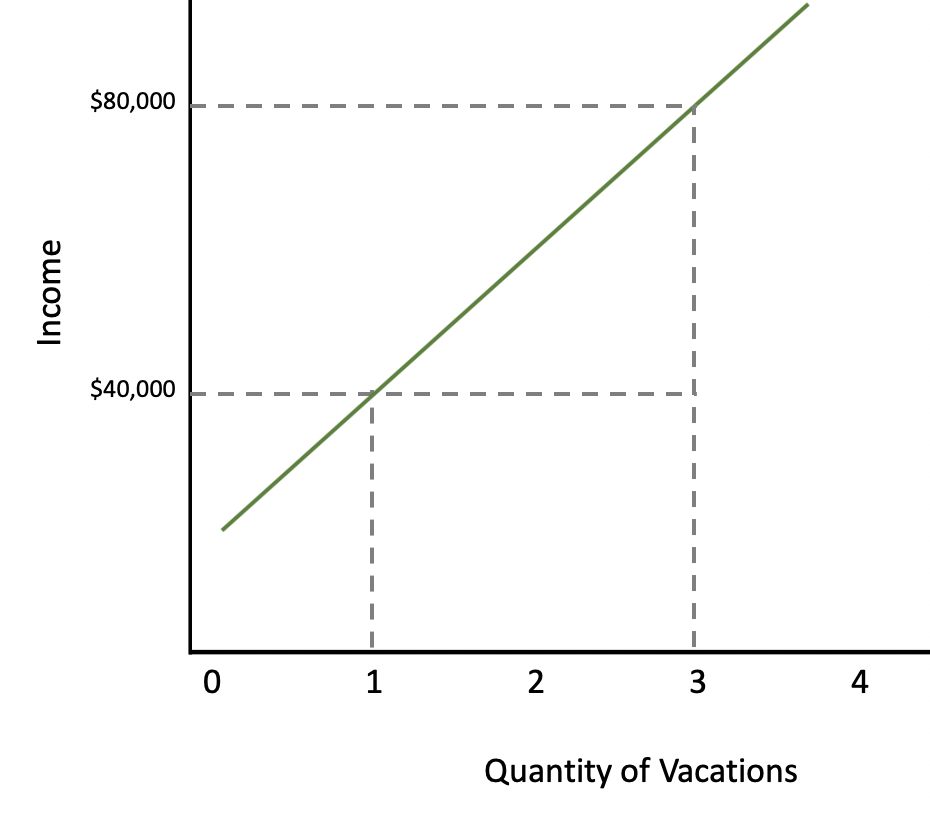Let's plug our numbers into the elasticity equation.The coefficient, if you plug the numbers in, would be a positive 2, which would indicate that vacations are a normal good.

term to know
Normal Goods
Goods for which demand increases as income increases

4b. Luxury Goods
However, vacations are also considered a luxury good. When coefficients are greater than 1, it means that our consumption of these goods increase by a greater percentage than the income increase. In this example, we were only able to afford one vacation before the income change.

A luxury good defined is a good that offers better quality and features which is consumed when income rises.

term to know
Luxury Goods
A good that offers better quality and features which is consumed when income rises

4c. Inferior Goods
Lastly, generic cereal is an example of an inferior good because you can see that as our income goes up, the quantity purchased goes down.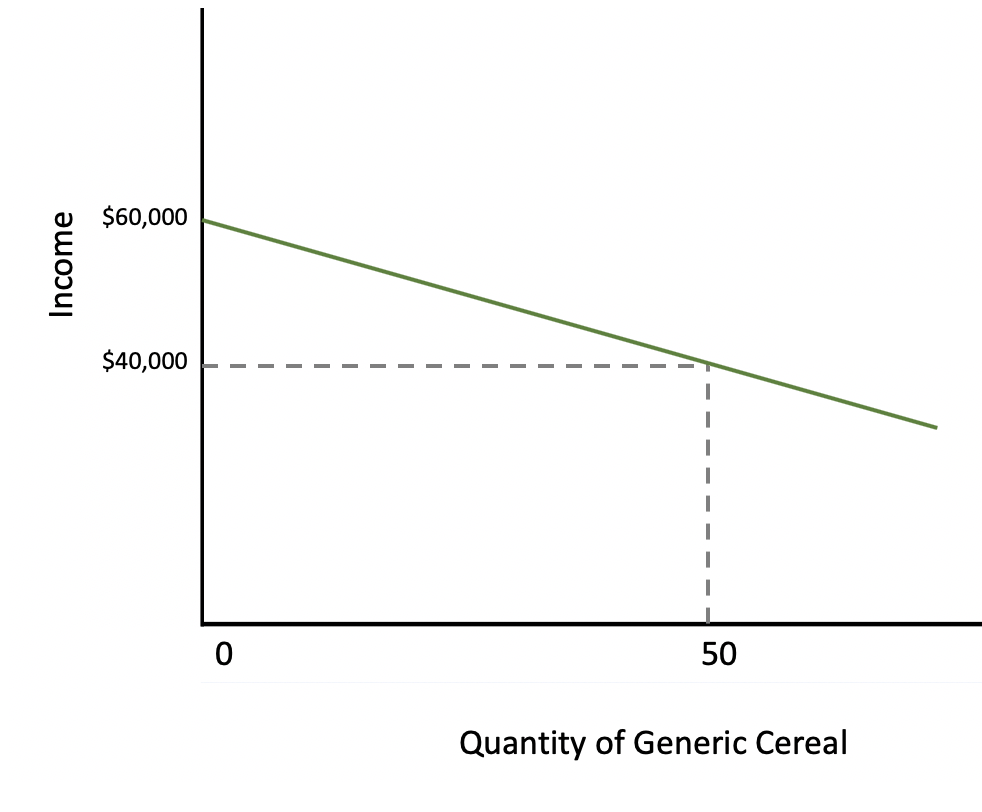For the above example, let's plug our numbers into the elasticity equation.The coefficient is negative, which indicates an inferior good, or one for which demand decreases as income increases.

term to know
Inferior Goods
Goods for which demand decreases as income increases

Income Elasticity Coefficients
E < 0 Inferior Good
E > 0 Normal Good
E > 1 Luxury Good

summary
Today we learned that elasticity measures how responsive consumers and producers are to a price change of a good itself or of a related good, and how responsive consumers are to an income change. We learned about the differences between perfectly inelastic, inelastic, perfectly elastic, elastic, and unit elastic. Lastly, we learned about three different types of elasticity: own-price elasticity, cross-price elasticity, and income elasticity, exploring examples of normal goods, luxury goods, and inferior goods.

Source: Adapted from Sophia instructor Kate Eskra.

Terms to Know
Cross-Price Elasticity

Change of demand (supply) that occurs due to change in price of substitutes or complements.

Elasticity

Measurement of change in quantity demanded (supplied) which indicates the sensitivity of one variable to characteristics of another variable or income.

Income Elasticity

An economic measure of change in relation to income and demand for normal, inferior, and luxury goods.

Inferior Goods

Goods for which demand decreases as income increases.

Luxury Goods

A good that offers better quality and features which is consumed when income rises.

Normal Goods

Goods for which demand increases as income increases.

Own-Price Elasticity

Elasticity of demand (supply) when the price of a good changes, keeping all other variables and goods separate.

Rating